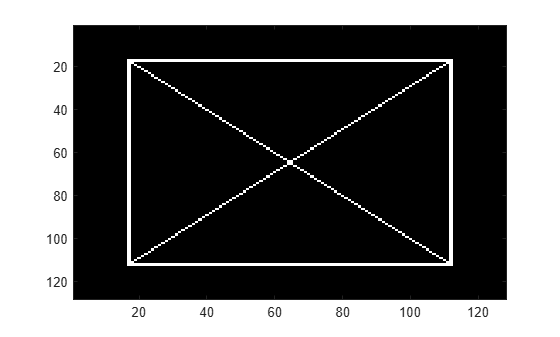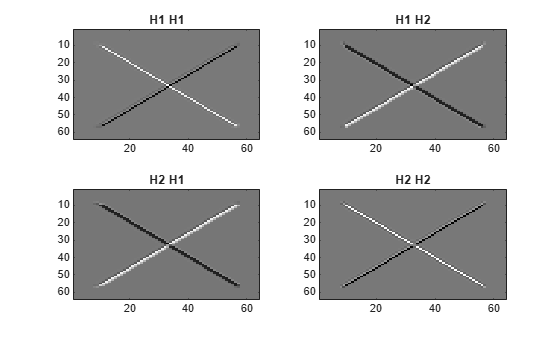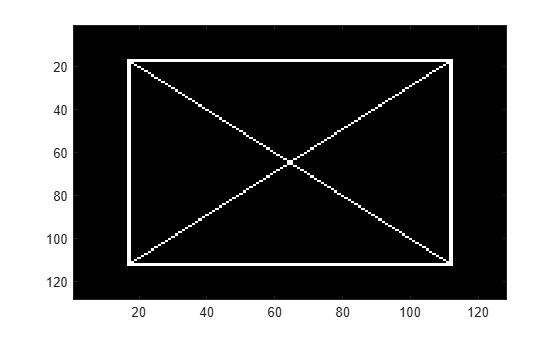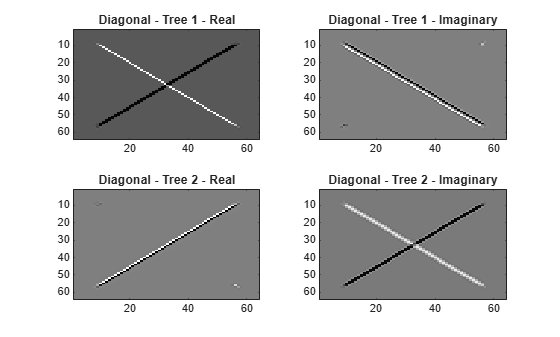dddtree2

Dual-tree and double-density 2-D wavelet transform

Description

example

wt = dddtree2(typetree,x,level,fdf,df) returns the typetree discrete wavelet transform of the 2-D input image, x, down to level, level. The wavelet transform uses the decomposition (analysis) filters, fdf, for the first level and the analysis filters, df, for subsequent levels. Supported wavelet transforms are the critically sampled DWT, double-density, real oriented dual-tree, complex oriented dual-tree, real oriented dual-tree double-density, and complex oriented dual-tree double-density wavelet transform. The critically sampled DWT is a filter bank decomposition in an orthogonal or biorthogonal basis (nonredundant). The other wavelet transforms are oversampled filter banks with differing degrees of directional selectivity.

example

wt = dddtree2(typetree,x,level,fname) uses the filters specified by fname to obtain the wavelet transform. Valid filter specifications depend on the type of wavelet transform. See dtfilters for details.

example

wt = dddtree2(typetree,x,level,fname1,fname2) uses the filters specified in fname1 for the first stage of the dual-tree wavelet transform and the filters specified in fname2 for subsequent stages of the dual-tree wavelet transform. Specifying different filters for stage 1 is valid and necessary only when typetree is 'realdt', 'cplxdt', 'realdddt', or 'cplxdddt'.

Examples

collapse all

Visualize the six directional wavelets of the real oriented dual-tree wavelet transform.

Create the first-stage Farras analysis filters for the two trees.

Faf{1} = [0      0
-0.0884   -0.0112
0.0884    0.0112
0.6959    0.0884
0.6959    0.0884
0.0884   -0.6959
-0.0884    0.6959
0.0112   -0.0884
0.0112   -0.0884
0         0];
Faf{2} = [ 0.0112  0
0.0112         0
-0.0884   -0.0884
0.0884   -0.0884
0.6959    0.6959
0.6959   -0.6959
0.0884    0.0884
-0.0884    0.0884
0    0.0112
0   -0.0112];

Create the 6-tap Kingsbury Q-shift analysis filters for subsequent stages of the multiresolution analysis.

af{1} = [ 0.0352   0
0         0
-0.0883   -0.1143
0.2339         0
0.7603    0.5875
0.5875   -0.7603
0    0.2339
-0.1143    0.0883
0         0
0   -0.0352];

af{2} = [0   -0.0352
0         0
-0.1143    0.0883
0    0.2339
0.5875   -0.7603
0.7603    0.5875
0.2339         0
-0.0883   -0.1143
0         0
0.0352         0];

To visualize the six directional wavelets, you will modify the wavelet coefficients of a four level real oriented dual-tree wavelet transform of an image of zeros. Create an image of zeros whose size satisfies the following constraints:

• The row and column dimensions are divisible by ${2}^{4}$.

• The minimum of the row and column size must be greater than or equal to the product of the maximum length of the analysis filters and ${2}^{3}$.

J = 4;
L = 3*2^(J+1);
N = L/2^J;
x = zeros(2*L,3*L);
[numrows,numcols] = size(x)
numrows = 192
numcols = 288

Obtain the real oriented dual-tree wavelet transform of the image of zeros down to level 4.

wt = dddtree2('realdt',x,J,Faf,af)
wt = struct with fields:
type: 'realdt'
level: 4
filters: [1x1 struct]
cfs: {1x5 cell}
sizes: [14x2 double]

The fourth element in wt.cfs are the level 4 wavelet coefficients. Insert a 1 in one position of the six wavelet subbands (three orientations $×$ two trees) at the coarsest scale, and invert the wavelet transform.

wt.cfs{4}(N/2,N/2+0*N,1,1) = 1;
wt.cfs{4}(N/2,N/2+1*N,2,1) = 1;
wt.cfs{4}(N/2,N/2+2*N,3,1) = 1;
wt.cfs{4}(N/2+N,N/2+0*N,1,2) = 1;
wt.cfs{4}(N/2+N,N/2+1*N,2,2) = 1;
wt.cfs{4}(N/2+N,N/2+2*N,3,2) = 1;
xrec = idddtree2(wt);

Visualize the six directional wavelets.

imagesc(xrec);
colormap gray; axis off;
title('Real Oriented Dual-Tree Wavelets')Obtain the double-density wavelet transform of an image.

Load the image and obtain the double-density wavelet transform using 6-tap filters (see dtfilters).

imagesc(xbox)
colormap graywt = dddtree2('ddt',xbox,1,'filters1')
wt = struct with fields:
type: 'ddt'
level: 1
filters: [1x1 struct]
cfs: {[64x64x8 double]  [64x64 double]}
sizes: [10x2 double]

In the critically sampled 2-D discrete wavelet transform, there is one highpass filter. Filtering the rows and columns of the image with the highpass filter corresponds to extracting details in the diagonal orientation. In the double-density wavelet transform, there are two highpass filters, H1 and H2. Diagonally oriented details are extracted by filtering the image rows and columns with four combinations of the highpass filters. Visualize the diagonal details in the four wavelet highpass-highpass subbands.

H1H1 = wt.cfs{1}(:,:,4);
H1H2 = wt.cfs{1}(:,:,5);
H2H1 = wt.cfs{1}(:,:,7);
H2H2 = wt.cfs{1}(:,:,8);
subplot(2,2,1)
imagesc(H1H1);
title('H1 H1')
colormap gray;
subplot(2,2,2);
imagesc(H1H2);
title('H1 H2')
subplot(2,2,3)
imagesc(H2H1)
title('H2 H1')
subplot(2,2,4)
imagesc(H2H2)
title('H2 H2')Obtain the complex dual-tree wavelet transform of an image. Show that the complex dual-tree wavelet transform can detect the two different diagonal directions.

Load the image and obtain the complex dual-tree wavelet transform.

imagesc(xbox)
colormap graywt = dddtree2('cplxdt',xbox,1,'FSfarras','qshift10')
wt = struct with fields:
type: 'cplxdt'
level: 1
filters: [1x1 struct]
cfs: {[5-D double]  [64x64x2x2 double]}
sizes: [5x2 double]

Obtain and display the diagonally oriented details from the two trees.

waveletcfs = wt.cfs{1};
subplot(2,2,1)
imagesc(waveletcfs(:,:,3,1,1))
title('Diagonal - Tree 1 - Real')
colormap gray
subplot(2,2,2)
imagesc(waveletcfs(:,:,3,1,2))
title('Diagonal - Tree 1 - Imaginary')
subplot(2,2,3)
imagesc(waveletcfs(:,:,3,2,1))
title('Diagonal - Tree 2 - Real')
subplot(2,2,4)
imagesc(waveletcfs(:,:,3,2,2))
title('Diagonal - Tree 2 - Imaginary')Input Arguments

collapse all

Type of wavelet decomposition, specified as one of 'dwt', 'ddt', 'realdt', 'cplxdt', 'realdddt', or 'cplxdddt'. The type, 'dwt', produces a critically sampled (nonredundant) discrete wavelet transform. The other decomposition types produce oversampled wavelet transforms. 'ddt' produces a double-density wavelet transform with one scaling and two wavelet filters for both row and column filtering. The double-density wavelet transform uses the same filters at all stages. 'realdt' and 'cplxdt' produce oriented dual-tree wavelet transforms consisting of two and four separable wavelet transforms. 'realdddt' and 'cplxdddt' produce double-density dual-tree wavelet transforms. The dual-tree wavelet transforms use different filters for the first stage (level).

Input image, specified as a matrix with even-length row and column dimensions. Both the row and column dimensions must be divisible by 2L, where L is the level of the wavelet transform. Additionally, the minimum of the row and column dimensions of the image must be greater than or equal to the product of the maximum length of the decomposition (analysis) filters and 2(L-1).

Data Types: double

Level of the wavelet decomposition, specified as a positive integer. If L is the value of level, 2L must divide both the row and column dimensions of x. Additionally, the minimum of the row and column dimensions of the image must be greater than or equal to the product of the maximum length of the decomposition (analysis) filters and 2(L-1).

The level-one analysis filters, specified as a matrix or cell array of matrices. Specify fdf as a matrix when typetree is 'dwt' or 'ddt'. The size and structure of the matrix depend on the typetree input as follows:

• 'dwt' — This is the critically sampled discrete wavelet transform. In this case, fdf is a two-column matrix with the lowpass (scaling) filter in the first column and the highpass (wavelet) filter in the second column.

• 'ddt' — This is the double-density wavelet transform. The double-density DWT is a three-channel perfect reconstruction filter bank. fdf is a three-column matrix with the lowpass (scaling) filter in the first column and the two highpass (wavelet) filters in the second and third columns. In the double-density wavelet transform, the single lowpass and two highpass filters constitute a three-channel perfect reconstruction filter bank. This is equivalent to the three filters forming a tight frame. You cannot arbitrarily choose the two wavelet filters in the double-density DWT. The three filters together must form a tight frame.

Specify fdf as a 1-by-2 cell array of matrices when typetree is a dual-tree transform, 'realdt', 'cplxdt', 'realdddt', or 'cplxdddt'. The size and structure of the matrix elements in the cell array depend on the typetree input as follows:

• For the dual-tree complex wavelet transforms, 'realdt' and 'cplxdt', fdf{1} is an N-by-2 matrix containing the lowpass (scaling) and highpass (wavelet) filters for the first tree and fdf{2} is an N-by-2 matrix containing the lowpass (scaling) and highpass (wavelet) filters for the second tree.

• For the double-density dual-tree complex wavelet transforms, 'realdddt' and 'cplxdddt', fdf{1} is an N-by-3 matrix containing the lowpass (scaling) and two highpass (wavelet) filters for the first tree and fdf{2} is an N-by-3 matrix containing the lowpass (scaling) and two highpass (wavelet) filters for the second tree.

Analysis filters for levels > 1, specified as a matrix or cell array of matrices. Specify df as a matrix when typetree is 'dwt' or 'ddt'. The size and structure of the matrix depend on the typetree input as follows:

• 'dwt' — This is the critically sampled discrete wavelet transform. In this case, df is a two-column matrix with the lowpass (scaling) filter in the first column and the highpass (wavelet) filter in the second column. For the critically sampled orthogonal or biorthogonal DWT, the filters in df and fdf must be identical.

• 'ddt' — This is the double-density wavelet transform. The double-density DWT is a three-channel perfect reconstruction filter bank. df is a three-column matrix with the lowpass (scaling) filter in the first column and the two highpass (wavelet) filters in the second and third columns. In the double-density wavelet transform, the single lowpass and two highpass filters constitute a three-channel perfect reconstruction filter bank. This is equivalent to the three filters forming a tight frame. For the double-density DWT, the filters in df and fdf must be identical.

Specify df as a 1-by-2 cell array of matrices when typetree is a dual-tree transform, 'realdt', 'cplxdt', 'realdddt', or 'cplxdddt'. For dual-tree transforms, the filters in fdf and df must be different. The size and structure of the matrix elements in the cell array depend on the typetree input as follows:

• For the dual-tree wavelet transforms, 'realdt' and 'cplxdt', df{1} is an N-by-2 matrix containing the lowpass (scaling) and highpass (wavelet) filters for the first tree and df{2} is an N-by-2 matrix containing the lowpass (scaling) and highpass (wavelet) filters for the second tree.

• For the double-density dual-tree complex wavelet transforms, 'realdddt' and 'cplxdddt', df{1} is an N-by-3 matrix containing the lowpass (scaling) and two highpass (wavelet) filters for the first tree and df{2} is an N-by-3 matrix containing the lowpass (scaling) and two highpass (wavelet) filters for the second tree.

Filter name, specified as a character vector or string scalar. For the critically sampled DWT, specify any valid orthogonal or biorthogonal wavelet filter. See wfilters for details. For the redundant wavelet transforms, see dtfilters for valid filter names.

First-stage filter name, specified as a character vector or string scalar. Specifying a first-level filter that is different from the wavelet and scaling filters in subsequent levels is valid and necessary only with the dual-tree wavelet transforms, 'realdt', 'cplxdt', 'realdddt', and 'cplxdddt'.

Filter name for stages > 1, specified as a character vector or string scalar. Specifying a different filter for stages > 1 is valid and necessary only with the dual-tree wavelet transforms, 'realdt', 'cplxdt', 'realdddt', and 'cplxdddt'.

Output Arguments

collapse all

Wavelet transform, returned as a structure with these fields:

Type of wavelet decomposition used in the analysis returned as one of 'dwt', 'ddt', 'realdt', 'cplxdt', 'realdddt', or 'cplxdddt'. 'dwt' is the critically sampled DWT. 'ddt' produces a double-density wavelet transform with one scaling and two wavelet filters for both row and column filtering. 'realdt' and 'cplxdt' produce oriented dual-tree wavelet transforms consisting of 2 and 4 separable wavelet transforms. 'realdddt' and 'cplxdddt' produce double-density dual-tree wavelet transforms consisting of two and four separable wavelet transforms.

Level of wavelet decomposition, returned as a positive integer.

Decomposition (analysis) and reconstruction (synthesis) filters, returned as a structure with these fields:

First-stage analysis filters, returned as an N-by-2 or N-by-3 matrix for single-tree wavelet transforms, or a 1-by-2 cell array of two N-by-2 or N-by-3 matrices for dual-tree wavelet transforms. The matrices are N-by-3 for the double-density wavelet transforms. For an N-by-2 matrix, the first column of the matrix is the scaling (lowpass) filter and the second column is the wavelet (highpass) filter. For an N-by-3 matrix, the first column of the matrix is the scaling (lowpass) filter and the second and third columns are the wavelet (highpass) filters. For the dual-tree transforms, each element of the cell array contains the first-stage analysis filters for the corresponding tree.

Analysis filters for levels > 1, returned as an N-by-2 or N-by-3 matrix for single-tree wavelet transforms, or a 1-by-2 cell array of two N-by-2 or N-by-3 matrices for dual-tree wavelet transforms. The matrices are N-by-3 for the double-density wavelet transforms. For an N-by-2 matrix, the first column of the matrix is the scaling (lowpass) filter and the second column is the wavelet (highpass) filter. For an N-by-3 matrix, the first column of the matrix is the scaling (lowpass) filter and the second and third columns are the wavelet (highpass) filters. For the dual-tree transforms, each element of the cell array contains the analysis filters for the corresponding tree.

First-level reconstruction filters, returned as an N-by-2 or N-by-3 matrix for single-tree wavelet transforms, or a 1-by-2 cell array of two N-by-2 or N-by-3 matrices for dual-tree wavelet transforms. The matrices are N-by-3 for the double-density wavelet transforms. For an N-by-2 matrix, the first column of the matrix is the scaling (lowpass) filter and the second column is the wavelet (highpass) filter. For an N-by-3 matrix, the first column of the matrix is the scaling (lowpass) filter and the second and third columns are the wavelet (highpass) filters. For the dual-tree transforms, each element of the cell array contains the first-stage synthesis filters for the corresponding tree.

Reconstruction filters for levels > 1, returned as an N-by-2 or N-by-3 matrix for single-tree wavelet transforms, or a 1-by-2 cell array of two N-by-2 or N-by-3 matrices for dual-tree wavelet transforms. The matrices are N-by-3 for the double-density wavelet transforms. For an N-by-2 matrix, the first column of the matrix is the scaling (lowpass) filter and the second column is the wavelet (highpass) filter. For an N-by-3 matrix, the first column of the matrix is the scaling (lowpass) filter and the second and third columns are the wavelet (highpass) filters. For the dual-tree transforms, each element of the cell array contains the first-stage analysis filters for the corresponding tree.

Wavelet transform coefficients, specified as a 1-by-(level+1) cell array of matrices. The size and structure of the matrix elements of the cell array depend on the type of wavelet transform, typetree as follows:

• 'dwt'cfs{j}(:,:,d)

• j = 1,2,... level is the level.

• d = 1,2,3 is the orientation.

• cfs{level+1}(:,:) are the lowpass, or scaling, coefficients.

• 'ddt'cfs{j}(:,:,d)

• j = 1,2,... level is the level.

• d = 1,2,3,4,5,6,7,8 is the orientation.

• cfs{level+1}(:,:) are the lowpass, or scaling, coefficients.

• 'realdt'cfs{j}(:,:,d,k)

• j = 1,2,... level is the level.

• d = 1,2,3 is the orientation.

• k = 1,2 is the wavelet transform tree.

• cfs{level+1}(:,:,k) are the lowpass, or scaling, coefficients.

• 'cplxdt'cfs{j}(:,:,d,k,m)

• j = 1,2,... level is the level.

• d = 1,2,3 is the orientation.

• k = 1,2 is the wavelet transform tree.

• m = 1,2 are the real and imaginary parts.

• cfs{level+1}(:,:,k,m) are the lowpass, or scaling, coefficients.

• 'realdddt'cfs{j}(:,:,d,k)

• j = 1,2,... level is the level.

• d = 1,2,3,4,5,6,7,8 is the orientation.

• k = 1,2 is the wavelet transform tree.

• cfs{level+1}(:,:,k) are the lowpass, or scaling, coefficients.

• 'cplxdddt'cfs{j}(:,:,d,k,m)

• j = 1,2,... level is the level.

• d = 1,2,3,4,5,6,7,8 is the orientation.

• k = 1,2 is the wavelet transform tree.

• m = 1,2 are the real and imaginary parts.

• cfs{level+1}(:,:,k,m) are the lowpass, or scaling, coefficients.

Each orientation corresponds to a particular subband. The double-density transforms 'ddt', 'realddt', and 'cplxdddt' generate wavelet coefficients of eight orientations. The other transforms, 'dwt', 'realdt', and 'cplxdt' generate wavelet coefficients of three orientations. The correspondence to subbands is as follows.

typetreeOrientations
'dwt', 'realdt', 'cplxdt'

'L' and 'H', denote the lowpass and highpass filters, respectively.

• d=1: 'LH' subband

• d=2: 'HL' subband

• d=3: 'HH' subband

'ddt', 'realdddt', 'cplxdddt'

'Lo', 'H1', and 'H2' denote the lowpass and two highpass filters, respectively.

• d=1: 'Lo H1' subband

• d=2: 'Lo H2' subband

• d=3: 'H1 Lo' subband

• d=4: 'H1 H1' subband

• d=5: 'H1 H2' subband

• d=6: 'H2 Lo' subband

• d=7: 'H2 H1' subband

• d=8: 'H2 H2' subband

Sizes of components in cfs, returned as an N-by-2 integer-valued matrix. The value of N depends on the level of wavelet decomposition and the type of wavelet decomposition: N = 2 + level × (number of orientations).

• cfs(1,:) = dimensions of input image.

• cfs(2+level,:) = dimensions of scaling coefficients.

• cfs(1+no×(i–1)+(1:no),:) = dimensions of level i detail coefficients, where no is the number of orientations.

Introduced in R2013b

Wavelet Toolbox DocumentationGet trial now